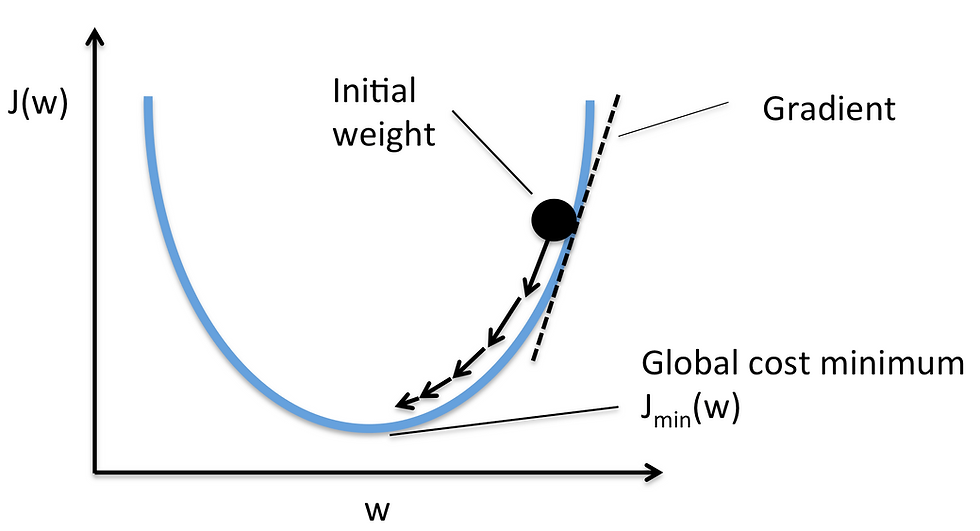top of page
Search
• Aristides Zenonos

# Gradient Descent vs. Normal Equation for Linear Regression problems

Updated: Jun 26, 2019Gradient descent gives one way of minimizing J. "Normal equation" performs the minimization explicitly and without resorting to an iterative algorithm. In the "Normal Equation" method, we will minimize J by explicitly taking its derivatives with respect to the θj ’s, and setting them to zero. This allows us to find the optimum theta without iteration. The normal equation formula is given below:

Pros:

O(kn^2)

Works well when n is large

Cons:

Need to choose alpha

Needs many iterations

Normal Equation:

Pros:

No need to choose alpha

No need to iterate

Cons:

O(n^3) need to calculate the inverse of X^(T)X

Slow if n is very large

Note:

With the normal equation, computing the inversion has complexity (n^3). So if we have a very large number of features, the normal equation will be slow. In practice, when n exceeds 10,000 it might be a good time to go from a normal solution to an iterative process. If X^(T)X is noninvertible, the common causes might be having :

• Redundant features, where two features are very closely related (i.e. they are linearly dependent)

• Too many features (e.g. m ≤ n). In this case, delete some features or use "regularization".

Source:

Andrew NG

https://www.coursera.org/learn/machine-learning/supplement/66bi5/normal-equation-noninvertibility